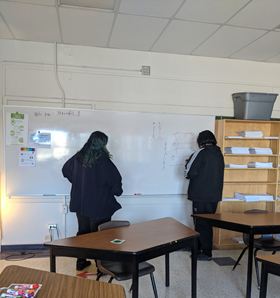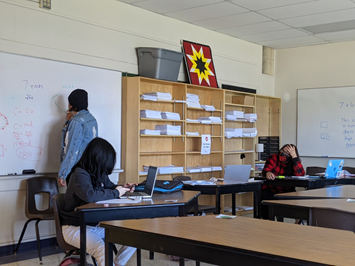# Math

November 3, 2022Argyle offers all three math courses for students to obtain their math credit at grade levels 10-12: Essential Math, Applied Math and Pre-Calculus MathEssential Mathematics

Essential Mathematics is intended for students whose post-secondary planning does not include a focus on mathematics and science-related fields.   Essential mathematics emphasizes the areas of consumer applications, problem solving, decision making and special sense.Applied Mathematics

Applied Mathematics is intended for students considering post-secondary studies who do not require a study of theoretical calculus.  It is context-driven and promotes the learning of numerical and geometrical problem-solving techniques as they relate to the world around us.

Primary goals of Applied Mathematics are to have students develop critical-thinking skills through problem solving and model real-world situations mathematically to make predictions.

Pre-Calculus MathematicsPre-Calculus Mathematics is designed for students who intend to study calculus and related mathematics as a part of post-secondary education.  Pre-Calculus Mathematics provides background knowledge and skills for the study of calculus in post-secondary institutions.

The course comprises of a high-level study of theoretical mathematics with an emphasis on problem solving and mental mathematics.